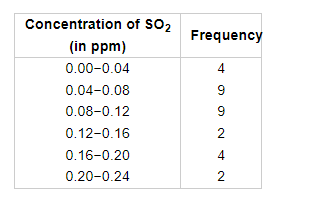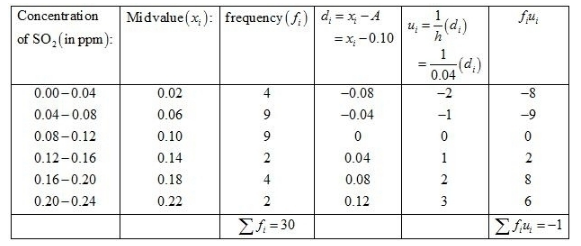# To find out the concentration of SO2 in the air (in parts per million, i.e., ppm),

Question:

To find out the concentration of $\mathrm{SO}_{2}$ in the air (in parts per million, i.e., ppm), the data was collected for 30 localities in a certain city and is presented below.Find the mean of concentration of $\mathrm{SO}_{2}$ in the air.

Solution:

Let the assumed mean A = 0.1 and h = 0.04.We know that mean, $\bar{X}=A+h\left(\frac{1}{N} \sum f_{i} u_{i}\right)$

Now, we have $N=\sum f_{i}=30, \sum f_{i} u_{i}=-1, h=0.04$ and $A=0.10$.

Putting the values in the above formula, we have

$\bar{X}=A+h\left(\frac{1}{N} \sum f_{i} u_{i}\right)$

$=0.10+0.04\left[\frac{1}{30} \times(-1)\right]$

$=0.10-\frac{0.04}{30}$

$=0.10-0.001$

$=0.099$

Hence, the mean concentration of $\mathrm{SO}_{2}$ in the air is $0.099 \mathrm{ppm}$.